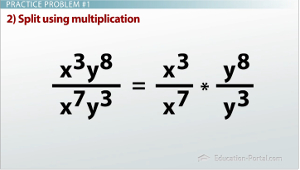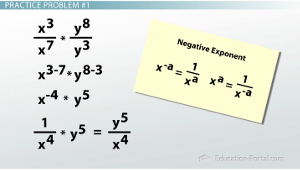# How to Simplify Expressions with Exponents

An error occurred trying to load this video.

Try refreshing the page, or contact customer support.

Coming up next: Rational Exponents

### You're on a roll. Keep up the good work!

Replay
Your next lesson will play in 10 seconds
• 0:05 Review of the…
• 0:29 Practice Problem #1
• 2:15 Practice Problem #2
• 3:15 Practice Problem #3

Want to watch this again later?

Timeline
Autoplay
Autoplay
Speed

#### Recommended Lessons and Courses for You

Lesson Transcript
Instructor: Kathryn Maloney

Kathryn teaches college math. She holds a master's degree in Learning and Technology.

In this video, we will put all of the exponent properties we have learned together. Don't let lots of numbers and letters confuse you! Simplifying exponents can be easy and fun!

## Review of the Properties of Exponents

Let's review the exponent properties:

If you're having problems memorizing these properties, I suggest using flash cards. Flash cards are a fantastic and easy way to memorize topics, especially math properties.## Practice Problem #1

So, let's get started!

Simplify is the same as reducing to lowest terms when we talk about fractions. Simplifying these terms using positive exponents makes it even easier for us to read.

Our first expression has x^3y^8 / y^3x^7. The first step I like to do is put the like terms on top of each other. On the top, I have x^3y^8. In the denominator, I want the xs over each other and the ys over each other, so I write x^7y^3.

My next step is to split these up using multiplication. This step is important when you first begin because you can see exactly what we are doing. Splitting the multiplication gives us x^3 / x^7 times y^8 / y^3.

Next step - look at each part individually. Since we have x^3 divided by x^7, we subtract their exponents. This gives us x^3-7. Since we have y^8 divided by y^3, we subtract their exponents. This gives us y^8-3. This will give us x^3-7, which is -4 and y^8-3, which is 5.Remember, we're simplifying using positive exponents, so we need to change x^-4. We know from our exponent properties that x^-4 is 1 / x^4 times y^5. Well, 5 is positive, so we don't need to change it.

My last step is to multiply. Our final, simplified answer is y^5 / x^4. This is our simplified answer with positive exponents.

## Practice Problem #2

Let me show you another one. This time we have 5x^2y^9 / 15y^9x^4. Let's rewrite this with like terms over each other: 5/15 times x^2 / x^4 times y^9/y^9

To unlock this lesson you must be a Study.com Member.

### Register to view this lesson

Are you a student or a teacher?

### Unlock Your Education

#### See for yourself why 30 million people use Study.com

##### Become a Study.com member and start learning now.
Back
What teachers are saying about Study.com

### Earning College Credit

Did you know… We have over 160 college courses that prepare you to earn credit by exam that is accepted by over 1,500 colleges and universities. You can test out of the first two years of college and save thousands off your degree. Anyone can earn credit-by-exam regardless of age or education level.